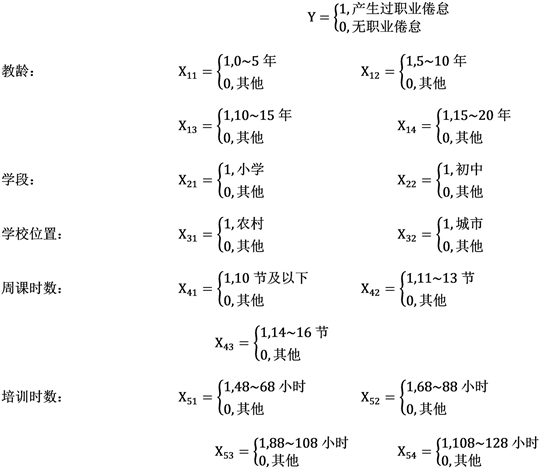# 杭州市中小学教师压力现状及对策研究A Study on the Current Situation of Pressure on Primary and Middle School Teachers in Hangzhou and the Strategies to Deal with It

DOI: 10.12677/SA.2020.93038, PDF, HTML, XML, 下载: 52  浏览: 129

Abstract: This paper takes Hangzhou primary and middle school teachers as the survey group. The purpose of this study is to investigate the current situation of the group’s stress and to study the effective decompression measures. First of all, we use simple random sampling to obtain survey data, and use words and charts to describe the basic information and situation. Then, we mine association rules for the status quo of pressure and the factors affected by pressure, and analyze the degree and source of pressure in a contingency table to find the correlation between them. Thirdly, we use principal component analysis to reduce the dimension of influencing factors, use AHP to determine the weight of factors, and analyze the influencing factors of pressure. Through the binary logistic regression model, this paper makes a regression analysis of “whether teachers have burnout emotion”. Finally, the influence of stress factors on teacher satisfaction is studied, and the relevant stress reduction measures are studied according to the weight of each factor.

1. 引言

2. 样本量的确定

${N}_{1}=\frac{{t}^{2}{s}^{2}}{{\text{e}}^{2}}=\frac{{t}^{2}{s}^{2}}{{r}^{2}{\stackrel{¯}{x}}^{2}}=543$

${N}_{2}={N}_{1}\frac{x}{x+{N}_{1}}=543×\frac{72000}{72000+543}=539$

$N=\frac{{N}_{2}}{r}=\frac{543}{85%}=674$

3. 教师压力影响因素调查结果分析

3.1. 基于主成分分析的影响因素降维

Step 1：取样适当性检验Table 1. The test of KMO and Bartlet

Step 2：提取因子Table 2. Explanation table of total variance of principal component analysis

Step 3：对各个因子进行分析Table 3. Load matrix after rotation

3.2. 基于层次分析确定因素权值

$A=\left(\begin{array}{cccc}1& 2& 3& 4\\ \frac{1}{2}& 1& 1& 2\\ \frac{1}{3}& 1& 1& 2\\ \frac{1}{4}& \frac{1}{2}& \frac{1}{2}& 1\end{array}\right)$Table 4. The weight of each factor in the criterion layer of AHP

$\left\{\begin{array}{l}{Y}_{1}=0.60{X}_{1}+0.40{X}_{4}\hfill \\ {Y}_{2}=0.52{X}_{7}+0.48{X}_{8}\hfill \\ {Y}_{3}=0.31{X}_{5}+0.38{X}_{6}+0.31{X}_{9}\hfill \\ {Y}_{4}=0.27{X}_{2}+0.73{X}_{3}\hfill \end{array}$

${Y}_{n}=\frac{{Y}_{n}权值×{Y}_{n}得分}{Z}$$n=1,2,3,4$。其中，评价值越接近1表明影响程度越大，越接近0表示影响程度越小。

4. 基于二元Logistic回归对压力与职业倦怠的研究

4.1. 模型的选取与变量的选取Table 5. Pearson chi square test of factors and burnoutFigure 1. Corresponding graph of dependent variable and independent variable

4.2. 模型的建立与求解

$\begin{array}{l}\mathrm{ln}\left(\frac{p}{1-p}\right)=23.32-1.02{X}_{11}-0.54{X}_{12}+0.34{X}_{13}-2.00{X}_{14}-1.09{X}_{21}-0.21{X}_{22}-1.42{X}_{31}\\ \text{\hspace{0.17em}}\text{\hspace{0.17em}}\text{\hspace{0.17em}}\text{\hspace{0.17em}}\text{\hspace{0.17em}}\text{\hspace{0.17em}}\text{\hspace{0.17em}}\text{\hspace{0.17em}}\text{\hspace{0.17em}}\text{\hspace{0.17em}}\text{\hspace{0.17em}}\text{\hspace{0.17em}}\text{\hspace{0.17em}}\text{\hspace{0.17em}}\text{\hspace{0.17em}}-2.09{X}_{32}-0.83{X}_{41}+0.35{X}_{42}+1.48{X}_{43}-20.47{X}_{51}-21.30{X}_{52}-21.63{X}_{53}-21.64{X}_{54}\end{array}$

4.3. 模型的应用Table 7. Burnout rate of typical teachers

5. 建议

5.1. 经济方面

5.2. 绩效方面

5.3. 教师个人方面

5.4. 社会地位

6. 结束语

  陈德云. 教师压力分析及解决策略[J]. 外国教育研究, 2002(12): 53-56.  李雪凤, 刘焕芳. 中职教师职业压力与倦怠的对比研究[J]. 山西财经大学学报, 2013, 35(S2): 80-81.  李琼, 张国礼, 周钧. 中小学教师的职业压力源研究[J]. 心理发展与教育, 2011, 27(1): 97-104.  欧阳偶春, 雷淑华. 中学教师职业压力研究述评[J]. 教学与管理, 2012(15): 57-58.  黄秀海. 高校教师压力情况的因子分析——以浙江省下沙高教园区为个例[J]. 现代教育科学, 2008(2): 68-72.  张华龙, 刘新华. 中小学教师评价研究的梳理与反思[J]. 现代教育科学. 2010(1): 125-128.  陈德云. 我国中小学教师压力来源分析与压力应对策略[J]. 江西教育科研, 2007(4): 45-48.  万雅静, 马文静, 张弛. 缓解教师压力的思考与建议[J]. 职业时空, 2007, 3(7): 55.